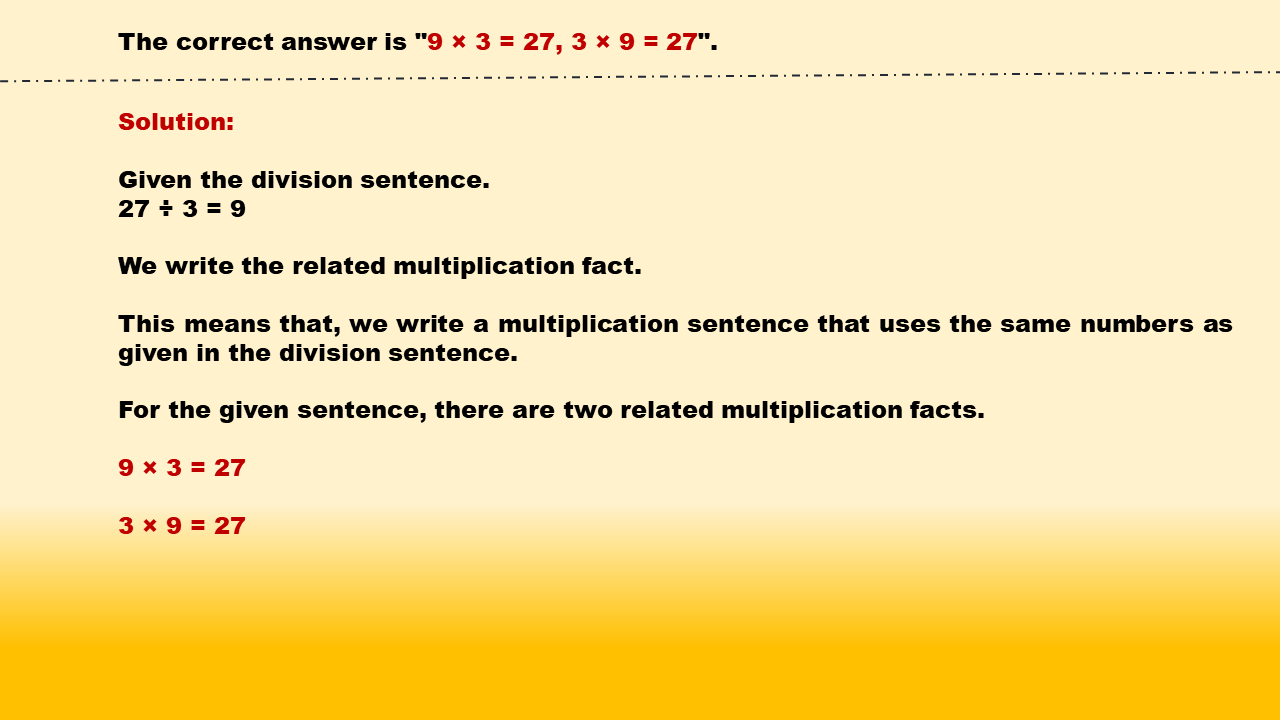1
visibility

Identify related multiplication facts for 27 ÷ 3 = 9.

• 9 × 3 = 27, 3 × 9 = 27

• 9 × 7 = 63, 7 × 9 = 63

• 9 × 5 = 45, 5 × 9 = 45

• 9 × 8 = 72, 8 × 9 = 72